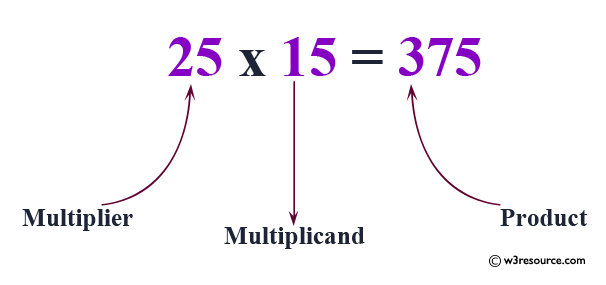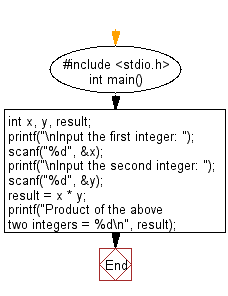﻿ C Program: Calculate the product of the two integers - w3resource

# C Exercises: Calculate the product of the two integers

## C Basic Declarations and Expressions: Exercise-10 with Solution

Write a C program that accepts two integers from the user and calculates the product of the two integers.

Pictorial Presentation:C Code:

``````#include <stdio.h>

int main()
{
int x, y, result; // Declare variables for two integers and their product

// Prompt user for input and store in 'x'
printf("\nInput the first integer: ");
scanf("%d", &x);

// Prompt user for input and store in 'y'
printf("\nInput the second integer: ");
scanf("%d", &y);

result = x * y; // Calculate the product of 'x' and 'y'

// Print the product
printf("Product of the above two integers = %d\n", result);
}
``````

Sample Output:

```Input the first integer: 25

Input the second integer: 15
Product of the above two integers = 375
```

Flowchart:C Programming Code Editor:

What is the difficulty level of this exercise?

Test your Programming skills with w3resource's quiz.

﻿